• 一些专业的定义或定理可以对事物的规律进行简洁的描述，揭示规律的本质，因此，随着对机器人运动学研究的深入，很有必要温习一下运动学的相关定义及定理。 基础定义 定义2.1：一个系统（system）是空间 X\mathbf XX...
机器人学–运动学的相关定义及定理一些专业的定义或定理可以对事物的规律进行简洁的描述，揭示规律的本质，因此，随着对机器人运动学研究的深入，很有必要温习一下运动学的相关定义及定理。
基础定义
定义2.1：一个系统（system）是空间 $\mathbf X$中的一个点集。
定义2.2：一个系统的位形（configuration）是指系统中各点的位置。
定义2.3：位形空间（configuration）是给定系统的全部位形组成的一个度量空间。
定义2.4：一个系统的自由度（degrees of freedom）是指其位形空间的维度。（一个不太精确但大致相当的定义是：用于确定一个位形所需的最少实数数目）。
定义2.5 ：位移（displacement）是指位形的变化，该变化并不会改变系统中任意两点间的距离，也不会改变系统的手性。
定义2.6：刚体（rigid body）是指仅会产生位移的一个系统。
定义2.7：转动（也称旋转，rotation）是指至少有一个固定点的位移。
定义2.8：平移（translation）是所有点沿平行线移动相同距离的一个位移。
定理2.1：带有函数组合（functional composition）的位移，构成一个群（group）。
定理2.2：对于欧式空间$\mathbf E^2$或$\mathbf E^3$中的任何位移$D$以及任意一点$O$，该位移$D$均可表述为一个平移和一个关于点$O$的旋转的叠加。
推论2.1：给定任意一点$O$,任何微分运动或速度都可以被分解为一个平移部分以及一个关于点$O$的旋转部分。
平面运动学
定理2.3：一个平面位移可以由任意两点的运动来完全确定。
该定理有很多应用，例如相机捕捉物体做平面位移时，可以通过定位物体上两个marker点，确定物体的运动。最近一个项目中要对工件安装在平台上的误差进行补偿，那么首先要识别出物体的平动和旋转，那么可以根据该定理来实现。
定理2.4：任何一个平面位移要么是平移，要么是旋转。
定理2.4另一种描述：每一个平面运动都是关于投影平面中一点的旋转。
推论2.2：将其推广到微分运动（即速度），每个平面速度都是关于投影平面内一个中心点的角速度。
瞬心极迹
任何平面运动的路劲都可通过平面内的两条曲线来描述，这些曲线被称为瞬心极迹（centrode）。其中的一条曲线，被称为瞬心极 迹，它沿着另一条被称为固定瞬心迹迹的曲线做无滑动的纯滚动。瞬心极迹提供了一种对平面运动的规范描述。
固定瞬心极迹是指旋转极点在固定平面内的轨迹图（locus）。运动瞬心极迹则是值旋转极点在运动平面内的轨迹图。随着运动瞬心极迹在固定瞬心极迹上做无滑动的纯滚动，两条曲线的接触点即为瞬心。
球面运动学
球面运动是指在一个球面上所有可能的运动。
定理2.5 ：给定球面上的两个点，如果它们不是对极点（antipodal）,那么球面上的位移可以通过这两点的运动而完全确定。
定理2.6（欧拉定理）：对于每个空间旋转，存在一条由对你的固定点组成的直线。换而言之，关于一点的任何旋转都是关于一条直线的旋转，该直线被称为旋转轴线（rotation axis）。
空间运动学
空间运动指的是空间中任意可能的运动。
螺旋位移（screw displacement）：绕空间中的某条轴线旋转，同时沿该条轴线平移。
定理2.7（Chasles theorem）：每个空间位移都是由关于某条轴线的旋转以及沿同一轴线的平移叠加而构成的。
定义2.9：旋量（screw）是空间中带有相关旋距（pitch）的一条线。其中，选举时指旋量的线性分量与角度分量之间的比率。
定义2.10：运动旋量（twist）是指旋量外加一个标量幅值，它给出了旋量轴的一个旋转加上沿旋量轴的一个平移。旋转角度为运动旋量的幅值大小，而平移距离则等于该幅值与选举的乘积。因此，旋距是平移与旋转之间的比率。
运动学约束
操作通常会涉及接触，而接触通常可以从运动学约束（kinematic constraint）方面进行建模，运动学约束是指对物体可能运动的约束。
对于一个动态约束（运动着的约束），称为非定常约束（rheonomic）约束，固定约束称为定常（scleronomic）约束

完整约束
可表述为$F(q,t)=0$形式的约束，该约束仅与位形变量或许还与时间有关，但与速度向量无关

不完整约束
无法表述为$F(q,t)=0$形式的约束，需要使用不等式或速度变量

定常约束
一个静态约束，形如$F(q,\dot q)=0$, 该约束与实践无关

非定常约束
一个动态约束，形如$F(q,\dot q,t)=0$,该约束涉及时间

令$\mathbf C$表示位形空间，同时将位形写为$\mathbf q\in\mathbf C$的形式。$\mathbf{T_qC}$为$\mathbf q$处的切空间(tangent space)，它由速度向量组成的空间，可被看作是$R^n$的原点被置于$\mathbf q$处时的拷贝。一个速度向量可被写作$\dot q\in\mathbf{T_qC}$。
定义2.11：$k$个Pfaffian约束组成的集合具有如下形式：
$\mathbf {w_i(q)\dot q}=0,i=1,2,\ldots,k$
其中，$\mathbf w_i$是线性无关的行向量，而$\dot q$是一个列向量。
定义2.12：向量场（vector field）是从位形$\mathbf q$到速度向量$\dot{ \mathbf q}$的一个光滑映射，如下：
$f(\mathbf q):\mathbf {C\to T_qC}$
定义2.13：分布（distribution）是指一个光滑映射，该映射为$\mathbf C$中的每个位形$\mathbf q$分配了一个线性子空间。
假设位形空间$\mathbf C$的维度为$n$，给定$k$个$Pfafian$约束，在任何位形 $\mathbf q$处，存在一个$(n-k)$维的可行速度线性子空间。
定义2.14：如果一个分布的维度在其位形空间内保持不变，则其是规则的（regular）。
定义2.15：令$f$和$g$为$C$内的两个向量场。定义里括号$[f,g]$为如下形式的向量场
$\frac{\partial g}{\partial q}f-\frac{\partial f}{\partial q}g$
李括号定义中的两个偏导数，它们是向量场相对于位形空间变化的导数，表示为$n\times n$的矩阵。
定义2.16：如果一个分布在李括号下是闭合的，则它是对合的（involutive）。
定义2.17：一个分布$\Delta$对合闭包（involutive closure）是该分布在李括号运算下的闭包$\overline \Delta$。
定理2.8（Frobenius定理）：一个规则分布是可积的，当且仅当该分布是一个对合分布。
参考文献
volutive closure）是该分布在李括号运算下的闭包$\overline \Delta$。
定理2.8（Frobenius定理）：一个规则分布是可积的，当且仅当该分布是一个对合分布。
参考文献
机器人操作中的力学原理[M]，Matthew T. Masson 著，贾振中，万伟伟译. 北京：机械工业出版社，2017.11


展开全文• 旋转矩阵是机器人运动学的基础，但有时候经常会被各种旋转求解问题搞晕，比如旋转向量、旋转坐标系、同一空间点在不同坐标系下的坐标求解等等。正好当前需要对机械臂进行行为规划，所以又重新学习并理解了一下旋转...

旋转矩阵是机器人运动学的基础，但有时候经常会被各种旋转求解问题搞晕，比如旋转向量、旋转坐标系、同一空间点在不同坐标系下的坐标求解等等。正好当前需要对机械臂进行行为规划，所以又重新学习并理解了一下旋转矩阵，特此记录一下此次重温的结果。

文章目录1. 本质理解2. 基础概念2.1 位置2.2 姿态3. 特例分析3.1 向量的表示3.2 向量的旋转4. 感性总结5. 参考文献
1. 本质理解
从本质上来理解，不管是坐标系中的向量旋转，还是同一空间点在不同坐标系的坐标求解等等，其最本质的原因都是坐标系之间的变换。所谓万变不离其宗，只要抓住了其本质属性，就不会再随便地被各种换着说法的求解问题搞晕。
因此在以下的介绍中会在不同的问题中寻找其共同点，总结出本质所在。
2. 基础概念
涉及到机器人运动学，就绕不开刚体的位姿这一概念，即刚体的位置和姿态。教材中的说明示意图如下图所示：2.1 位置
刚体可以由其在空间中相对于参考坐标系的位置和方向进行完整的描述。在上图中假设 $O-xyz$ 为标准正交参考坐标系，其中由蓝色箭头代表的$\boldsymbol{ x,y,z}$为坐标轴的单位向量。
则刚体上的点$O'$相对于坐标系$O-xyz$的位置可以表示为：$\boldsymbol o'=o'_x\boldsymbol x+o'_y\boldsymbol y+o'_z\boldsymbol z$ 其中$o'_x, o'_y, o'_z$表示向量$\boldsymbol o'$在参考坐标系的坐标轴上的分量。而$\boldsymbol o'$的位置写为(3X1)的向量为：$\boldsymbol o' = \begin{bmatrix} o'_x \\ o'_y \\ o'_z \end{bmatrix}$而这个向量的完整描述可以表述为基与坐标的乘积：$\boldsymbol o' = \begin{bmatrix} \boldsymbol{x\, y\, z} \end{bmatrix} \begin{bmatrix} o'_x \\ o'_y \\ o'_z \end{bmatrix}$
2.2 姿态
除了位置之外，还需要对刚体的指向，即姿态进行描述。而这也是旋转矩阵最本质的来源，即来源于 坐标系的旋转，这一部分理解不好或是理解不透彻，会导致此后面对各种形式的旋转时出现混乱，所以公式将进行详细的表述。
描述姿态的方式就是为刚体建立一个固连于刚体的标准正交坐标系，并由 其相对于参考坐标系的 单位向量 在参考坐标系中的描述 来表示。如上图所示，刚体的局部固连参考系为 $O-x'y'z'$ ，其局部固连参考系坐标系的单位向量为$\boldsymbol{ x',y',z'}$，而这局部坐标系的单位向量在参考坐标系 $O-xyz$ 中的表示为：$\boldsymbol x' = a_1\boldsymbol x + a_2\boldsymbol y + a_3\boldsymbol z \\ \boldsymbol y' = b_1\boldsymbol x + b_2\boldsymbol y + b_3\boldsymbol z \\ \boldsymbol z' = c_1\boldsymbol x + c_2\boldsymbol y + c_3\boldsymbol z$其中$a_1, a_2, a_3$分别表示局部参考坐标系的$x'$轴的基向量$\boldsymbol x'$在参考坐标系 $O-xyz$ 三个轴上的分量。其它的两轴同理。对于上式，可以进行进一步的整理，即上式等价于：$\boldsymbol{[x'\; y'\; z']} = \begin{bmatrix} \boldsymbol{x\; y\; z} \end{bmatrix} \begin{bmatrix} a_1\; b_1\; c_1 \\ a_2\; b_2\; c_2 \\ a_3\; b_3\; c_3 \end{bmatrix}$最后一个矩阵就是两个坐标系基之间的关系，即两个坐标系之间的转换关系，将这个矩阵定义为旋转矩阵$\boldsymbol R$。$\boldsymbol R = \begin{bmatrix} a_1\; b_1\; c_1 \\ a_2\; b_2\; c_2 \\ a_3\; b_3\; c_3 \end{bmatrix}$
通过计算过程也可以得出，当前这一旋转矩阵，是  $O-x'y'z'$ 坐标系相对于 $O-xyz$ 的，同时需要注意的也有：旋转矩阵的每一列表示的是对应的刚体坐标系的基在原参考坐标系下的表示，所以每一列之间是相互正交的，且模长为1，所以旋转矩阵是一个正交矩阵，并且符合以下性质：$\boldsymbol{R^TR=I} \\ \boldsymbol{R^T=R^{-1}}$第二个性质说明，旋转矩阵的转置与其逆矩阵相等。
3. 特例分析
3.1 向量的表示
向量的表示问题其实就是同一空间点在不同坐标系下的求解问题，为了理解其几何意义，暂时不考虑坐标系之间的位移，即假设所有相关坐标系的原点重合。考虑一个坐标系（称之为新坐标系）通过参考坐标系（称之为旧坐标系）相对于某一旋转轴旋转得到。如果相对旋转轴作逆时针方向旋转，则旋转方向为正，反之则为负。如下图所示：相对于旧坐标系 $O-xyz$，空间中的一点 $\boldsymbol P$ 可以表示为$\boldsymbol p = \begin{bmatrix} \boldsymbol{x\; y\; z} \end{bmatrix} \begin{bmatrix} p_x \\ p_y \\ p_z \end{bmatrix}$而相对于新坐标系 $O'-x'y'z'$,同一点可以表示为$\boldsymbol p' = \begin{bmatrix} \boldsymbol{x'\; y'\; z'} \end{bmatrix} \begin{bmatrix} p'_x \\ p'_y \\ p'_z \end{bmatrix}$而以上两个方程式之间的唯一联系就是新旧坐标系之间的联系，即代表坐标系的基之间的联系。所以将上式与之前推导的坐标系之间的关系联立可以得到：$\boldsymbol p' = \begin{bmatrix} \boldsymbol{x'\; y'\; z'} \end{bmatrix} \begin{bmatrix} p'_x \\ p'_y \\ p'_z \end{bmatrix} = \begin{bmatrix} \boldsymbol{x\; y\; z} \end{bmatrix} \begin{bmatrix} a_1\; b_1\; c_1 \\ a_2\; b_2\; c_2 \\ a_3\; b_3\; c_3 \end{bmatrix} \begin{bmatrix} p'_x \\ p'_y \\ p'_z \end{bmatrix}$因为$\boldsymbol{p, p'}$在空间中是同一个点，当然$\boldsymbol{p, p'}$的数值并不一样，但因为是空间中同一个点，所以如果将它们的坐标同时转换到同一个参考坐标系下，则数值必然是相等的，所以通过在同一基下的$\boldsymbol{p, p'}$的表示式，可以得到纯坐标的关系为：$\begin{bmatrix} p_x \\ p_y \\ p_z \end{bmatrix} = \begin{bmatrix} a_1\; b_1\; c_1 \\ a_2\; b_2\; c_2 \\ a_3\; b_3\; c_3 \end{bmatrix} \begin{bmatrix} p'_x \\ p'_y \\ p'_z \end{bmatrix}$ 即新坐标系中的点左乘新坐标系相对于旧坐标系的旋转矩阵就等于当前点在旧坐标系中的位置。 从矩阵关系上可以很容易得出同一个点在不同坐标系下的坐标转换关系。但正如之前在第一部分所讲到的，这所有的旋转形式，不管是同一空间点在不同坐标系下的坐标关系还是向量的旋转求解，其本质都是坐标系之间的变换。那么如何从几何上理解同一点在不同坐标系下的不同表示这一问题呢？
如果想清楚其中的关键，其实很简单，甚至顺便把向量旋转的问题也解决了，那么其中的关键点是什么？用下图辅助说明：假设存在一个 $\boldsymbol p_0$ 点，位于旧坐标系中，其在旧坐标系中的坐标在数值上与 $\boldsymbol p$ 点在新坐标系中的坐标完全相同。那么旧坐标系经过新坐标系相对于旧坐标系的旋转关系旋转之后， $\boldsymbol p_0$ 点与 $\boldsymbol p$ 点必然是重合的。所以同一空间点在不同坐标系下的坐标求解问题，本质上是新旧坐标系之间的旋转变换问题，是旧坐标系带着与新坐标系中的点具有相同坐标数据的属于旧坐标系的点进行了新坐标系相对于旧坐标系旋转关系的旋转变换之后，所得到的点在旧坐标系中的坐标表示问题。 而这个问题，同时也是向量旋转问题。通过以上分析，可得出同一空间点在不同坐标系下的坐标求解问题的本质，是坐标系间的相对变换关系。想清楚了这一部分的几何解释，再来看向量的旋转问题。
3.2 向量的旋转
如下图所示，教科书中讲：旋转矩阵也可视为使某一向量绕空间中任一轴旋转给定角度的矩阵算子。从图中也可以看出，向量$\boldsymbol p'$ 绕 $z$ 轴旋转 $\alpha$ 弧度之后，生成新的向量 $\boldsymbol p$。计算算式为 $\boldsymbol p = R_z(\alpha)\boldsymbol p'$。那从几何来说是为什么呢？其实这个例子比一个更直观，因为从几何上理解更容易一点，就是旧坐标系带着隶属于旧坐标系的向量$\boldsymbol p'$绕着旧坐标系的 $z$ 旋转了 $\alpha$ 弧度之后，将向量$\boldsymbol p'$绕到了现在向量 $\boldsymbol p$ 的位置，所以向量$\boldsymbol p$ 的新坐标中的坐标数值就是向量$\boldsymbol p'$在旧坐标系中的坐标数值。现在求解的就是这个随着旧坐标系到达新位置的向量$\boldsymbol p'$在之前的旧坐标中的位置。
所以在计算上，当然是 新坐标系中的点左乘新坐标系相对于旧坐标系的旋转矩阵就等于当前点在旧坐标系中的位置。
4. 感性总结
经过这一次对旋转矩阵的重新学习与理解，试着将旋转相关的问题都解释为坐标系间的变换，将各种具有不同表象的问题统统归因于坐标系间的相对变换，返本归源。理解上又透彻了一分。
5. 参考文献
 Bruno Siciliano, Lorenzo Sciavicco, Luigi Villani, Giuseppe Oriolo. Robotics:Modeling,Planning and Control[M]. Springer-Verlag London, 2009.


展开全文旋转矩阵
• 文章目录机器人学名词与概念解释位姿描述运动学自由度正运动学/逆运动学关节空间/笛卡尔空间/驱动器空间DH表示连杆参数连杆附加坐标系连杆变换矩阵灵巧工作空间/可达工作空间欧拉变换/RPY变换重复精度/定位精度奇异...
机器人学名词与概念解释

张文政
2019年12月31日

文章目录机器人学名词与概念解释位姿描述运动学自由度正运动学/逆运动学关节空间/笛卡尔空间/驱动器空间DH表示连杆参数连杆附加坐标系连杆变换矩阵灵巧工作空间/可达工作空间欧拉变换/RPY变换重复精度/定位精度奇异问题/退化问题代数解法/几何解法雅可比矩阵由雅可比矩阵给出的奇异性动力学基于能量的拉格朗日函数方法基于力平衡的牛顿-欧拉方程方法对比轨迹规划路径/轨迹路径规划/轨迹规划（来源于互联网）点到点轨迹规划/直线轨迹规划机器人控制标定/初始化传感器
位姿描述
位置和姿态，是三维空间中物体两个非常重要的特性。
几种对坐标系$B$的描述方式：

位置的描述用$3 \times 1$的位置矢量，即位置矢量可对世界坐标系中的任何点进行定位；姿态的描述用$3 \times 3$的旋转矩阵，即在物体上固定一个坐标系后给出的此坐标系相对于参考坐标系的表达。位置和姿态成对出现的组合（四个矢量）称为坐标系：$\{B\}=\{^A_BR, ^AP_{BORG}\}$

X-Y-Z固定角。首先将坐标系$\{B\}$和一个已知参考坐标系$\{A\}$重合。先将$\{B\}$绕$\hat{X}_A$旋转$\gamma$角（回转角），再绕$\hat{Y}_A$旋转$\beta$角（俯仰角），最后绕$\hat{Z}_A$旋转$\alpha$角（偏转角）。由于都是绕着一个固定的坐标系转，且顺序为XYZ，因此得名。
$^A_BR_{XYZ}(\gamma,\beta,\alpha)=R_Z(\alpha)R_Y(\beta)R_X(\gamma)$

Z-Y-X欧拉角。首先将坐标系$\{B\}$和一个已知参考坐标系$\{A\}$重合。先将$\{B\}$绕$\hat{Z}_B$旋转$\alpha$角，再绕$\hat{Y}_B$旋转$\beta$角，最后绕$\hat{X}_B$旋转$\gamma$角。每次旋转所绕的轴的方位取决于上次的旋转，这样三个一组的旋转被称作欧拉角。旋转顺序为ZYX，因此得名。
$^A_BR_{Z‘Y’X‘}=R_Z(\alpha)R_Y(\beta)R_X(\gamma)$

三者对比：

不同点

旋转矩阵
是一个正交矩阵，需要9个数字来表示一个姿态，确定姿态不是很方便

X-Y-Z固定角
顺序地绕固定参考坐标系的XYZ轴旋转，只需要输入三个角度即可

Z-Y-X欧拉角
绕运动坐标系的ZYX轴旋转，只需输入三个角度即可

运动学
运动学：研究物体的运动，而不考虑引起这种运动的力。运动学中研究位置、速度、加速度和位置变量对时间或其他变量的高阶微分。
自由度
操作臂自由度数目是指具有独立位置变量的数目。对于操作臂，关节数目等于自由度数目。
当一个操作臂少于6自由度时，它在三维空间内不能达到全部位姿。
这样就引出了欠驱动机器人与冗余机器人：
系统驱动器的数目小于或大于自由度的数目，则称为欠驱动或冗余。或者指控制输入小于/大于系统自由度的机器人。
正运动学/逆运动学
正运动学：给定一组关节角的值，计算工具坐标系相对于基坐标系的位姿。即从关节空间描述到笛卡尔空间描述的操作臂位置表示。
逆运动学：给定操作臂末端执行器的位姿，计算所有可达给定位姿的关节角。即将机器人位姿从笛卡尔空间向关节空间映射。需要考虑解的存在性、多重解性（PUMA机器人到达一个确定的目标有8个解）和解的求法（数值解法，封闭解（代数法、几何法））。
关节空间/笛卡尔空间/驱动器空间
关节空间：对于一个具有$n$个自由度的操作臂，它的所有连杆位置可由一组$n$个关节变量加以确定，这样的一组变量常被称为$n\times1$的关节矢量，所有关节矢量组成的空间称为关节空间。
笛卡尔空间：位置在空间相互正交的轴上测量，用三个变量描述空间一点的位置，用另外三个变量描述物体的姿态。有时称为任务空间或操作空间。
**驱动器空间：**机械臂由驱动器驱动，驱动器矢量组成的空间。
DH表示
用连杆参数描述机构运动关系的规则。
连杆参数
规定从操作臂的固定基座开始为连杆进行编号，称固定基座为连杆0，第一个可动连杆为连杆1，操作臂末端连杆为连杆$n$。机器人的每个连杆都可以用四个运动学参数即连杆参数描述（$\alpha_{i-1}, a_{i-1}, d_i, \theta_{i}$）。其中两个用于描述连杆本身（$a,\alpha$），两个用于描述连杆间的连接关系（关节变量$\theta, d$）。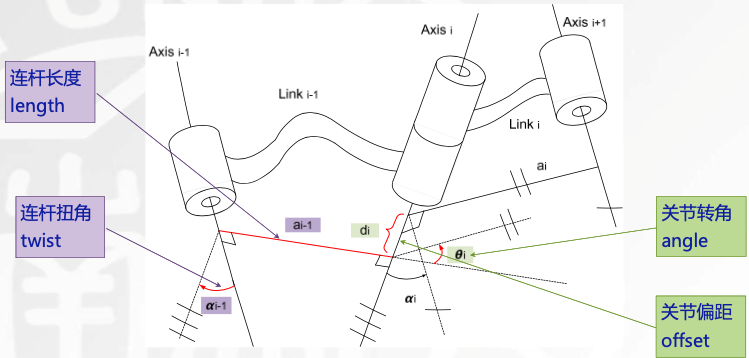连杆附加坐标系
在每个连杆上定义一个固连坐标系。固定在连杆$i$上的固连坐标系为坐标系$\{i\}$。在定义好参考坐标系$\{0\}$后（可以任意设定），按如下步骤建立坐标系：

找出关节轴，画出轴线延长线；
找出关节轴$i$和$i+1$之间 的公垂线或交点，以交点或公垂线与关节轴$i$的交点作为连杆坐标系$\{i\}$的原点；
$\hat{Z}_i$轴沿关节轴$i$的指向；
$\hat{X}_i$轴沿公垂线指向，若两轴相交，规定$\hat{X}_i$轴垂直于两轴所在平面（$X_i$垂直于$Z_iZ_{i+1}$）；
右手定则确定$\hat{Y}_i$的方向；
当第一个关节变量为0时，规定坐标系$\{0\}$和$\{1\}$重合。对于坐标系$\{N\}$，原点和$\hat{X}_N$轴方向可以任取，但尽量使连杆参数为0。

在建好坐标系后，连杆参数就可这样定义（绕两个X两个z，动两个Z两个X）：

$a_{i-1}$：绕$\hat{X}_{i-1}$轴，从$\hat{Z}_{i-1}$移动到$\hat{Z}_i$的距离；
$\alpha_{i-1}$：绕$\hat{X}_{i-1}$轴，从$\hat{Z}_{i-1}$旋转到$\hat{Z}_i$的角度（右手定则）；
$d_i$：绕$\hat{Z}_i$轴，从$\hat{X}_{i-1}$移动到$\hat{X}_i$的距离；
$\theta_i$：绕$\hat{Z}_i$轴，从$\hat{X}_{i-1}$旋转到$\hat{X}_i$的角度（右手定则）。

连杆变换矩阵
最终目标：求出连杆$n$相对于连杆$0$的位姿，$^{0}_{N}T$；
第一步分解：$^{0}_{N}T=^{0}_{1}T^{1}_{2}T^{2}_{3}T\cdots^{N-1}_{N}T$，相邻连杆间的坐标系变换，$\{i\}$相对于坐标系$\{i-1\}$的变换$^{i-1}_{i}T$；
第二步分解：引入三个中间坐标系$\{P\},\{Q\},\{R\}$分解成四个变换，$^{i-1}_{R}T,^{R}_{Q}T,^{Q}_{P}T,^{P}_{i}T$，每个变换都是仅有一个连杆参数的函数。使用平移算子$D_{Q}(\cdot)$和旋转算子$R_{K}(\cdot)$的定义方式，可得如下形式（其实也就是上一节确定四个连杆参数的方法对应到数学表达式，按$\alpha,a,\theta,d$的顺序）：
$^{i-1}_{i}T=R_X(\alpha_{i-1})D_X(a_{i-1})R_{Z}(\theta_i)D_Z(d_i)$
灵巧工作空间/可达工作空间
工作空间是指操作臂末端执行器（而非DH表示中最后关节坐标系的原点）所能到达的范围。决定了逆运动学解的存在性。
灵巧工作空间指末端执行器能够从各个方向到达的空间区域；灵巧系数=可达角度所占弧长/单位圆周长
可达工作空间是至少从一个方向上有一个方位可以到达的空间。显然，灵巧工作空间是可达工作空间的子集。
欧拉变换/RPY变换
基于绕腕坐标系确定腕的方位，从而可以确定机械手的方位。工业机器人中经常使用两种旋转角组合：

**欧拉变换：**欧拉角合成的齐次变换；
**RPY变换：**滚动（roll）、俯仰（pitch）、侧偏角（yaw）合成的齐次变换。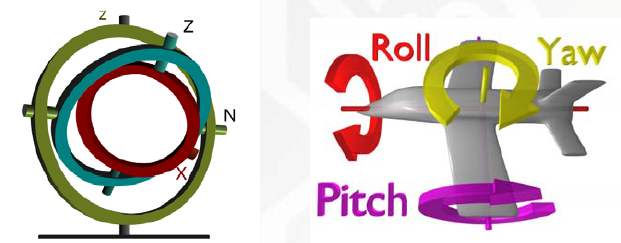重复精度/定位精度
**示教点：**操作臂运动实际到达的点，然后关节位置传感器读取关节角并存储；
计算点：对于可将目标位置描述为笛卡尔坐标系的系统，它可以将操作臂移动到工作空间中一个从未示教过的点，这种点叫计算点；
重复精度：关节空间中回到示教点，末端执行器在笛卡尔空间的精度；
定位精度：在笛卡尔空间内达到计算点的精度。
奇异问题/退化问题
当进行逆变换的计算时要做除法，而当分母趋于零时便会出现奇异现象。

工作空间边界的奇异位形：出现在操作臂完全展开或收回使得末端执行器处于或接近工作空间边界的情况；
工作空间内部的奇异位形：出现在远离工作空间边界，通常由于两个或两个以上的关节轴共线引起。

在求逆问题时可能出现多解现象，即同一操作机位姿对应于多于一组的关节变量的解存在，则手臂处于退化状态。显然这种不确定性的问题很容易解决。
代数解法/几何解法
代数解法：

列出DH参数表，用连杆变换矩阵求出机械臂运动学方程$^{0}_{N}T$；
根据机械臂的限制（例如平面机器人通过确定$x,y,\phi$）即可确定操作臂子空间；
用运动学方程描述的可达点必须在操作臂子空间内，列等式求解方程；

雅可比矩阵
定义了从关节空间速度（关节角速度$\dot{\Theta}$）向笛卡尔空间速度（一般研究末端执行器的线速度和角速度$\dot{x}=\left[\begin{array}{l} {v_{n}} \\ {\omega_{n}} \end{array}\right]$）的映射。雅可比矩阵实质上是一种时变的线性变换关系。
雅可比矩阵本身是多元形式的导数，是一个矢量对另一个矢量求导的结果。对于$m \times n$维空间的机器人（$m$是空间的维数，$n$是关节个数），雅可比矩阵为（当然，我们往往定义方阵形式的雅可比矩阵（分子布局），阶为关节个数）：
$J(\Theta)=\left[\begin{array}{cccc} {\frac{\partial f_{1}}{\partial \theta_{1}}} & {\frac{\partial f_{1}}{\partial \theta_{2}}} & {\cdots} & {\frac{\partial f_{1}}{\partial \theta_{n}}} \\ {\vdots} & {\vdots} & {\cdots} & {\vdots} \\ {\frac{\partial f_{m}}{\partial \theta_{1}}} & {\frac{\partial f_{m}}{\partial \theta_{2}}} & {\cdots} & {\frac{\partial f_{m}}{\partial \theta_{n}}} \end{array}\right]$
$\dot{x}=J(\Theta) \dot{\Theta}$
由雅可比矩阵给出的奇异性
如果雅可比矩阵可逆，则给定末端执行器速度，就可以求出相应的关节速度：
$\dot{\Theta}=J^{-1}(\Theta) \dot{x}$
但雅可比矩阵并非对所有的$\Theta$都可逆，奇异时，末端执行器位置称之为机械臂的奇异点。
机械臂位于奇异状态时，它在直角坐标空间的自由度就会减少。
动力学
动力学研究操作臂的运动和使之运动而施加的力和力矩之间的关系。操作臂动力学关注于两个问题：

已知一个轨迹点（robot motion）$\Theta, \dot\Theta,\ddot\Theta$，求期望的关节力矩矢量$\tau$。对控制很有用；
计算在施加一组关节力矩的情况下操作臂如何运动，即1的反过程。对仿真很有用。

基于能量的拉格朗日函数方法
操作臂的拉格朗日函数表示为：
$\mathcal{L}(\Theta,\dot\Theta)=k(\Theta,\dot\Theta)-u(\Theta)$
其中$k(\Theta,\dot\Theta)$表示系统的总动能，是关节位置和速度的标量函数；$u(\Theta)$是系统的总势能，是关节位置的标量函数。注意动能是系统的总动能，包括线速度与角速度；势能则包括重力势能和弹性势能。
因此拉格朗日-欧拉方程表示为：
$\frac{d}{d t}\left[\frac{\partial \mathcal L}{\partial \dot\Theta}\right]-\frac{\partial \mathcal L}{\partial \Theta}=\tau$
基于力平衡的牛顿-欧拉方程
两者都是描述了力、惯量和加速度之间的关系。

牛顿方程：面向平动，力平衡，$F=m \dot{v}$
欧拉方程：面向转动，力矩平衡，$J_{c} \dot{\omega}+\omega \times\left(J_{c} \omega\right)=\tau$

方法对比

拉格朗日欧拉方程：可以写成结构完美的形式，但如果不加以简化，则因计算困难而很难用于实际控制；
牛顿欧拉方程：给出了一组效率很高的递归方程，但很难用于推导出高级控制规律；
G-D运动方程：结构较好，计算速度高于拉格朗日，并且能把杆件平移和转动的效应明显的表示出来，有利于操作机的设计。

轨迹规划
路径/轨迹
路径是轨迹的空间几何形状。

**路径：**机器人位形的一个特定序列，不考虑时间因素；
**轨迹：**末端执行器在参考坐标系空间由初始点运动到终止点产生的空间曲线。与何时到达路径中的每个点有关，依赖速度和加速度，强调时间性。

路径规划/轨迹规划（来源于互联网）
两者都是运动规划。都是根据给定的任务起点（有时也包括中间点）对机器人建立运动方程，使其满足特定约束，并求解得到函数表达式或者数值点序列。
路径规划是找到一系列要经过的路径点，路径点是空间中的位置或关节角度，而轨迹规划是赋予路径时间信息。
路径规划的目标是使路径与障碍物的距离尽量远同时路径的长度尽量短；
轨迹规划的目的主要是机器人关节空间移动中使得机器人的运行时间尽可能短，或者能量尽可能小。
轨迹规划在路径规划的基础上加入时间序列信息，对机器人执行任务时的速度与加速度进行规划，以满足光滑性和速度可控性等要求。
点到点轨迹规划/直线轨迹规划
点到点的轨迹规划算法可以理解为在规定的时间$T$内，从已知关节空间起始点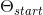运动到末尾点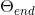的方法。
直线轨迹规划指让工具在相隔较远的中间点之间走直线。
机器人控制
标定/初始化
机器人初始化：找到自身当前的位置；
标定：找自身与作用环境之间的位置关系。
传感器
灵敏度评估的对象是传感器的输出和输入之间的关系。
分辨率是指传感器可感受到的被测量的最小变化的能力。描述刻度划分。
精度用来评估传感器测量系统测量精度。


展开全文• 光流的概念是Gibson在1950年首先提出来的。它是空间运动物体在观察成像平面上的像素运动的瞬时速度，是利用图像序列中像素在时间域上的变化以及相邻帧之间的相关性来找到上一帧跟当前帧之间存在的对应关系，从而计.....
注：本文参考了大连理工大学-硕士研究生：李世云的硕士学位论文（一种基于光流传感器的移动机器人定位方法），在对李世云先生表示衷心的感谢，如有错误，欢迎指正，谢谢！
1. 光流模块定位原理
**
光流的概念是Gibson在1950年首先提出来的。它是空间运动物体在观察成像平面上的像素运动的瞬时速度，是利用图像序列中像素在时间域上的变化以及相邻帧之间的相关性来找到上一帧跟当前帧之间存在的对应关系，从而计算出相邻帧之间物体的运动信息的一种方法。一般而言，光流是由于场景中前景目标本身的移动、相机的运动，或者两者的共同运动所产生的。
2. 为什么要使用光流模块？
**
通常移动机器人在进行定位导航时，主要依靠惯性导航元件IMU定向以及连接于轮组电机上的码盘定位来进行导航。理想环境下可以取得很好的效果，但是机器人遇到被卡住、缠住以及其他情况导致打滑时，机器人定位将出现较大误差，严重影响机器人地图构建的准确性，使得导航错误。相较而言，光流模块（PAA5101EW光流传感器模块（原相公司））不受轮组打滑的影响，能够弥补码盘测速的不足，实验也证明将光流模块加入移动机器人后，其能很大程度上减小由于轮组打滑带来的误差。
3. 运动学建模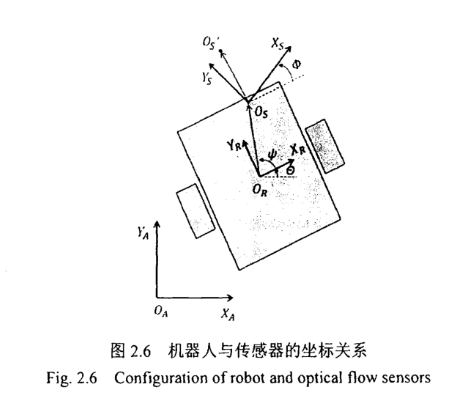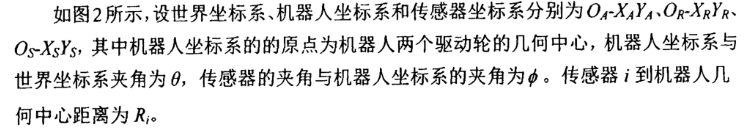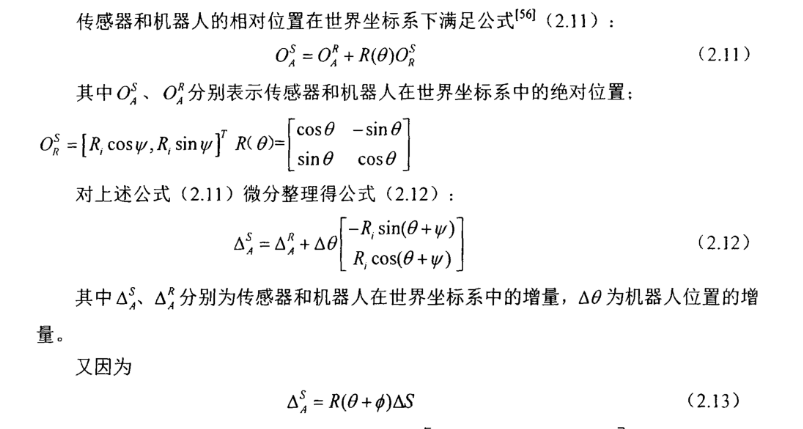其中$\Delta S=\begin{bmatrix} \\ Sx \\Sy \end{bmatrix}$，Sx为光流模块测量x轴向的增量，Sy为光流模块测量y轴方向的增量,
R($\theta+\phi)=\begin{bmatrix} &\\cos(\theta+\phi) &-sin(\theta+\phi) &\\sin(\theta+\phi) &cos(\theta+\phi) \end{bmatrix}$，注意：这里与原参考文献不一样，纠正了其中的一处错误。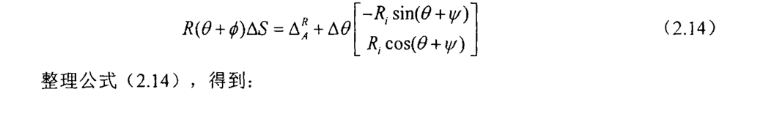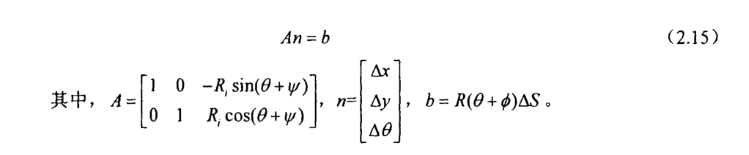进而可以得到：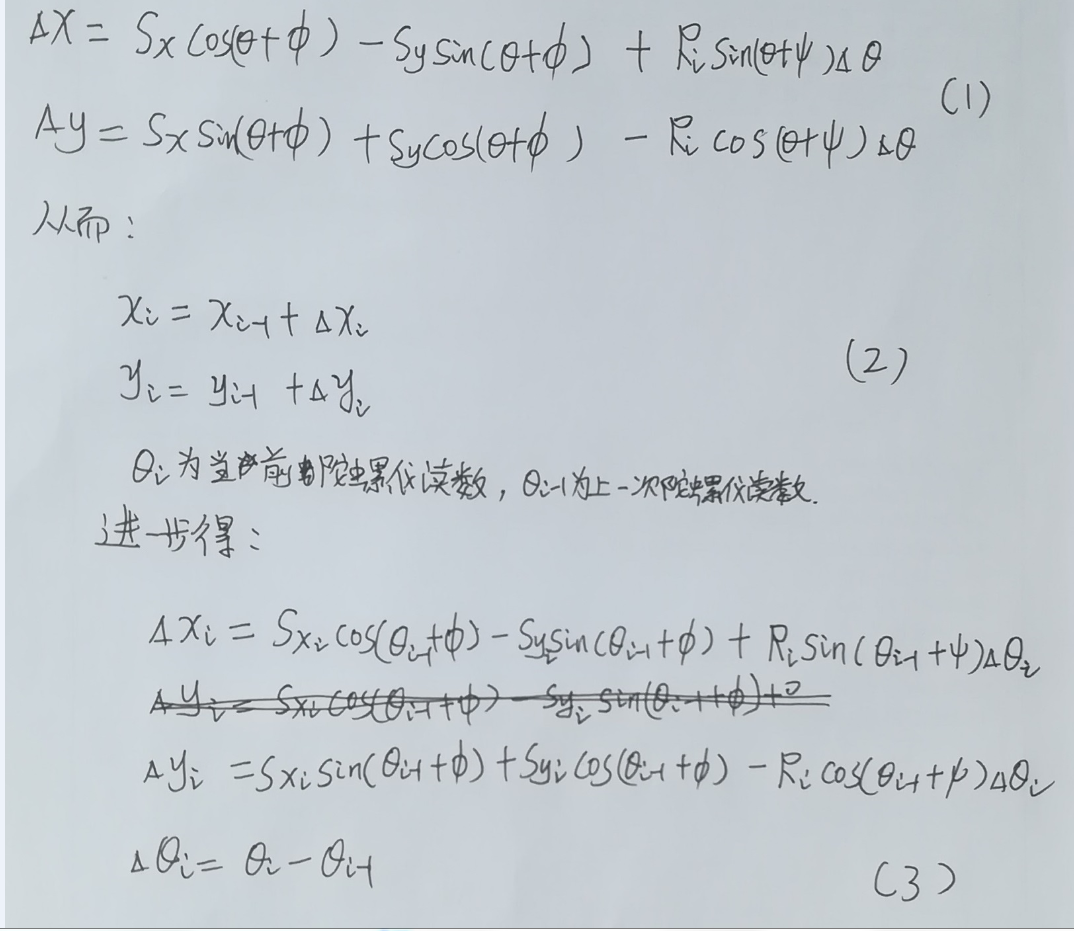最终我们需要使用的就是（2）和（3）式，通过不断的积分累加计算就可以的得到机器人当前的位置（xi, yi）。


展开全文定位
• 机器人运动学与动力学入门（一）自由度与连杆机构 在讨论机器人运动之前，先复习一些基本概念（啰里吧嗦环节）。已经了解童鞋可以自行跳过该节。 1.自由度（degree of freedom） 自由度描述一个物体可以自由...
• 以下内容为本人原创创作，转载请注明。谢谢 2.Rigid body motion 3. Rotation motion and its representations 4. Rigid body motion and its representations 5. Sum
• 模拟1：轨迹跟踪运动学优化 ##项目目标： RobotHAZMAT项目是一个由10位Virginia Tech高级机械工程师组成设计团队。 主要目标是为危险响应情况的机器人系统开发一种直观手势控制方法。 当前危险响应...MATLAB
• 通过引入瞬时作业点 的概念,将工件和刀具离散化,并用其相应坐标系上的一个向量来描述工件和刀具上的瞬时作业 点,建立了协作系统中各单机间的联系,将并联机器人协作运动学问题转化成二单并联机器人在 工件和刀具约束...
• 运动学的齐次变换矩阵T，是从关节的Θ\ThetaΘ推知末端执行器的笛卡尔坐标 雅克比矩阵0v=0J(Θ)Θ˙^0v=^0J(\Theta)\dot{\Theta}0v=0J(Θ)Θ˙，是从关节的Θ˙\dot\ThetaΘ˙速度推知末端执行器的速度（线速度+...
• 之前所做工作仅集中在 Rhino 机器人运动学程序模拟上。 因此，这项工作背后主要动机是能够执行运动学程序以及实时控制 Rhino 机器人。 两个图形用户界面，RhinoGui 和 RhinoWrite 是在 Matlab? 中开发。 ...matlab
• 整个流程主要两部分组成，前端Path Finding部分用来找出一条可行路径，后端Trajectory Optimization部分将可行路径优化成符合机器人运动学动力学模型一条轨迹用于机器人控制。可以看到在后端Trajectory ...
• 其中运动学逆解直接输出给舵机，控制机器人的运动，因此运动学逆解很重要。 2 基本概念 2.1机械结构模型   对于8自由度机器人，其机械结构模型如下图所示。图中两个并联大腿L1L_1L1​杆分别由独立舵机带动，假设...
• 运动学正/逆解概念 1.运动学正解:已知舵机/电机转角，求足端坐标。 2.运动学逆解:已知足端坐标，求舵机/电机转角。 二.足端轨迹规划 摆线方程: { x=r*(t-sint) y=r*(1-cost) [其中r为圆半径，t是圆半径所经过...
• 一味的啃机器人学的概念和公式枯燥又无味，坚持不了几天就从入门到放弃一条龙走完了。坚持下去的动力之一就是能尽快看到自己的学习成果，而MATLAB机器人工具箱就是非常简单的机器人平台快速搭建工具。有了它 ...matlab
• 主要涉及雅可比的概念，定义为机械臂末端笛卡尔速度与关节速度的线性变换，是一个偏导矩阵 https://wenku.baidu.com/view/03d586024afe04a1b171de8d.html51页起 以及...matlab
• Mario是一种运动学上很简单5自由度机械臂设置，我创建该机器人的目的是学习机器人的基本概念。 它最初是使用机械臂打开烤箱设计挑战。 但是，在实现该目标后不久，我开始将MARIO用作逆运动学，正向运动学和轨迹...
• 本教材以力学理论和控制理论全面讲述为特色。教材重点在于用严谨而系统方式介绍机器人动力学与控制基本概念和主要结果。全面介绍了机器人建模...全书共3章，分别是：机器人运动学，机器人动力学，机器人控制。
• 本文讨论的机器人，为空间开链连杆机构，其中的运动副（转动副或移动副）常称为关节，关节个数通常即为机器人的自由度数。 即：机器人由一系列关节（Joint）和连杆（Link）组成，这些关节可能是滑动（线性）或旋转...D-H坐标系
• 《机器人动力学与控制》霍伟，Word版，该教材详细介绍了不同控制方法动机、原理以及推导过程，对于设计机器人控制方法十分有用！...全书共3章，分别是：机器人运动学，机器人动力学，机器人控制。
• 从空间变换到正逆向运动学（一）前言空间变换的数学描述1.空间变换的基本概念2.旋转矩阵的性质3.几种常见的姿态描述方法4.小结 前言 一直有计划开个坑，写一个比较系统完整的博客专栏，一方面是有利于自己巩固所学的...
• 本教材以力学理论和控制理论全面讲述为特色。教材重点在于用严谨而系统方式介绍机器人动力学与控制基本概念和主要结果。全面介绍了机器人建模...全书共3章，分别是：机器人运动学，机器人动力学，机器人控制。
• 第2版提供了对相关领域内容的深入讲解，并仍保持独特的风格：它的重点不是集中在运动学机器人学的计算结果上，而是包含极其重要的最新材料，它们反映了这一领域的重要进展，并将机器人学与群论和几何学中的数学...
• 布朗大学和MIT研究团队正致力于开发一种系统，让机器人基于基本运动能力，形成对现实世界对象和想法抽象概念，从而规划任务。借助这个系统，机器人可以完成复杂任务，而不必纠缠于完成任务所需
• 入门材料： 基础内容中，个人认为最重要的必须掌握的...③雅克比矩阵：机械臂运动学的核心，用于关节速度和末端速度的换算； ④拉格朗日动力学：力和速度加速度之间换算的桥梁。 最重要的工具：数学，尤其是线性代数。
• 在正向运动学之前，我们需要知道如何在不同坐标系中表示向量。这时候就需要用到旋转矩阵定义了。 旋转矩阵有两个概念上但数学上等价解释。它们可以被看作是用其他坐标系表示一个坐标系中向量一种方法。...ROS 路径规划 人工智能
• 背景 motion planning, trajectory planning, path planning三者之间的关系。参考（看文献的时候经常看到...机器人运动规划中configuration space构形空间的概念。参考 (大神勿喷，本人EE背景，非ME、CS、ST...算法
• 戴建生编著的这本《机构学与机器人学的几何基础与旋量代数》起始于直线几何与线性代数，自然过渡到旋量代数与有限位移旋量，紧密联系李群、李代数、对偶数、Hamilton四元数、Clifford对偶四元数等现代数学基础，首次...
• 差动驱动运动学 状态机行为 基于测渗法的机器人定位 轨迹追踪控制器 解决方案代码在Python 3中提供。 如何使用 模拟实验室以一系列教程形式提供，其中包括对Webots官方教程引用。 从第一个实验开始，打算按顺序...
• Delta Robot – 适合学生的运动学联动机器人 这是一个小型Delta机器人。 它们通常用于装配线上取放作业。 它们在 Rostock/Kossel 风格 3D 打印机中也很常见。 这个机器人倒置和右侧朝上一样好用。 硬件 请...
• 引入基于旋量理论的运动旋量、力旋量及偏速度旋量等概念,推导得出五自由度上肢康复机器人的Kane动力方程,结果表明采用旋量理论分析机器人更加简明有效,比建立局部坐标系D-H法更简易。Kane方程求解只需加、减、...